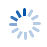Learn problem solving in Math by solving Math problems.## Solve Math Problems

Difficulty
Topic
Problem (1 of 1)
Listen
0:30 *
There are 650 hundred-peso bills. How much does a bundle have if we group the bills into 65 bundles?

Type ^2 instead of (²), ^3 for (³), ^deg for (°) in the answer.

* Easy problems must be solved mentally (ballpens up) for 10 seconds only. The average and hard problems have 30 seconds and 1 minute limits, respectively.

## Problem Solving Tips

You can't solve a Math problem if you can't add, subtract, multiply, or divide. Make sure that you have memorized the multiplication and addition tables up to 10x10 and 10+10.

Doing arithmetic calculations should be automatic and effortless. Subtraction and division are the opposite operations of addition and multiplication, respectively.

Follow the rule of precedence by doing multiplication and division first before addition and subtraction. The order is from left to right, but the parenthesized expression always takes priority.

### 2. Understand the Problem

Identify what is asked ("unknown") and which operation/s to use by carefully reading the problem. Establish the relationships that exist between the given values and the unknown.

Read through the problem again to ensure nothing is missed. Interpret the problem based on real-life situations. Rightly understood, a problem is considered half-solved.

### 3. Formulate a Solution

Use a standard formula if applicable, or create your own by translating the problem statement/s to mathematical sentence/s. Develop the simplest solution and avoid a complicated approach.

In some cases, there could be another unknown that may require a preliminary solution. Do not assume anything that is not given.

Perform the required operations step-by-step until you arrive at the answer. Use a uniform unit of measure for all the given values.

Simplify your answer to the lowest terms and, whenever applicable, don't forget to append the unit of measure used.

This is done by reversing the process. The answer becomes a given; then one of the given becomes the unknown.

Another way to check the answer is to redo the process, i.e., to solve the problem all over again. The answer should be the same; if not, review the process again.

As you do this, be mindful of the allowed time and try not to exceed it. Find the balance between speed and accuracy.

## Notes

### Common Prefixes

• micro 1/1000000
• milli 1/1000
• centi 1/100
• deci 1/10
• deca 10
• hecto 100
• kilo 1000
• mega 1000000
• bi 2
• tri 3
• penta 5
• hexa 6
• hepta 7
• octa 8
• nona 9

### Standard Units

• mm millimeter
• cm centimeter
• dm decimeter
• m meter
• km kilometer
• mg milligram
• g gram
• kg kilogram
• s second
• min minute
• hr hour
• l liter = 1 dm³ or 1,000 cm³
• ha hectare = 10,000 m²

### Formulas

Perimeter

• Triangle P = a+b+c
• Square P = 4s
• Rectangle P = 2(L+W)
• Circle C = 2πr or πD

Area

• Triangle A = 1/2bh
• Square A = s²
• Rectangle A = LW
• Trapezoid A = 1/2h(b1+b2)
• Circle A = πr²

Volume

• Cube V = s³
• Rectangular Prism V = LWH
• Circular Prism V = πr²h
• Prism (any) V = Ah, where A is area of the base
• Cone V = 1/3πr²h
• Sphere V = 4/3πr³
• Pyramid V = 1/3Ah, where A is area of the base

Simple Interest

• I = Prt

Sum of Consecutive Numbers (from n1 to n2)

• ∑ = 1/2(n1 + n2)(n2 - n1 + 1)

Sum of n Consecutive Even Numbers (starting from 2)

• ∑ = n(n+1)

Sum of n Consecutive Odd Numbers (starting from 1)

• ∑ = n²

Multiplication Table
 1 2 3 4 5 6 7 8 9 10 2 4 6 8 10 12 14 16 18 20 3 6 9 12 15 18 21 24 27 30 4 8 12 16 20 24 28 32 36 40 5 10 15 20 25 30 35 40 45 50 6 12 18 24 30 36 42 48 54 60 7 14 21 28 35 42 49 56 63 70 8 16 24 32 40 48 56 64 72 80 9 18 27 36 45 54 63 72 81 90 10 20 30 40 50 60 70 80 90 100